# sklearn.linear_model.LogisticRegression¶

class sklearn.linear_model.LogisticRegression(penalty='l2', dual=False, tol=0.0001, C=1.0, fit_intercept=True, intercept_scaling=1, class_weight=None, random_state=None, solver='warn', max_iter=100, multi_class='warn', verbose=0, warm_start=False, n_jobs=None)[source]

Logistic Regression (aka logit, MaxEnt) classifier.

In the multiclass case, the training algorithm uses the one-vs-rest (OvR) scheme if the ‘multi_class’ option is set to ‘ovr’, and uses the cross- entropy loss if the ‘multi_class’ option is set to ‘multinomial’. (Currently the ‘multinomial’ option is supported only by the ‘lbfgs’, ‘sag’ and ‘newton-cg’ solvers.)

This class implements regularized logistic regression using the ‘liblinear’ library, ‘newton-cg’, ‘sag’ and ‘lbfgs’ solvers. It can handle both dense and sparse input. Use C-ordered arrays or CSR matrices containing 64-bit floats for optimal performance; any other input format will be converted (and copied).

The ‘newton-cg’, ‘sag’, and ‘lbfgs’ solvers support only L2 regularization with primal formulation. The ‘liblinear’ solver supports both L1 and L2 regularization, with a dual formulation only for the L2 penalty.

Read more in the User Guide.

Parameters: penalty : str, ‘l1’ or ‘l2’, default: ‘l2’ Used to specify the norm used in the penalization. The ‘newton-cg’, ‘sag’ and ‘lbfgs’ solvers support only l2 penalties. New in version 0.19: l1 penalty with SAGA solver (allowing ‘multinomial’ + L1) dual : bool, default: False Dual or primal formulation. Dual formulation is only implemented for l2 penalty with liblinear solver. Prefer dual=False when n_samples > n_features. tol : float, default: 1e-4 Tolerance for stopping criteria. C : float, default: 1.0 Inverse of regularization strength; must be a positive float. Like in support vector machines, smaller values specify stronger regularization. fit_intercept : bool, default: True Specifies if a constant (a.k.a. bias or intercept) should be added to the decision function. intercept_scaling : float, default 1. Useful only when the solver ‘liblinear’ is used and self.fit_intercept is set to True. In this case, x becomes [x, self.intercept_scaling], i.e. a “synthetic” feature with constant value equal to intercept_scaling is appended to the instance vector. The intercept becomes intercept_scaling * synthetic_feature_weight. Note! the synthetic feature weight is subject to l1/l2 regularization as all other features. To lessen the effect of regularization on synthetic feature weight (and therefore on the intercept) intercept_scaling has to be increased. class_weight : dict or ‘balanced’, default: None Weights associated with classes in the form {class_label: weight}. If not given, all classes are supposed to have weight one. The “balanced” mode uses the values of y to automatically adjust weights inversely proportional to class frequencies in the input data as n_samples / (n_classes * np.bincount(y)). Note that these weights will be multiplied with sample_weight (passed through the fit method) if sample_weight is specified. New in version 0.17: class_weight=’balanced’ random_state : int, RandomState instance or None, optional, default: None The seed of the pseudo random number generator to use when shuffling the data. If int, random_state is the seed used by the random number generator; If RandomState instance, random_state is the random number generator; If None, the random number generator is the RandomState instance used by np.random. Used when solver == ‘sag’ or ‘liblinear’. solver : str, {‘newton-cg’, ‘lbfgs’, ‘liblinear’, ‘sag’, ‘saga’}, default: ‘liblinear’. Algorithm to use in the optimization problem. For small datasets, ‘liblinear’ is a good choice, whereas ‘sag’ and ‘saga’ are faster for large ones. For multiclass problems, only ‘newton-cg’, ‘sag’, ‘saga’ and ‘lbfgs’ handle multinomial loss; ‘liblinear’ is limited to one-versus-rest schemes. ‘newton-cg’, ‘lbfgs’ and ‘sag’ only handle L2 penalty, whereas ‘liblinear’ and ‘saga’ handle L1 penalty. Note that ‘sag’ and ‘saga’ fast convergence is only guaranteed on features with approximately the same scale. You can preprocess the data with a scaler from sklearn.preprocessing. New in version 0.17: Stochastic Average Gradient descent solver. New in version 0.19: SAGA solver. Changed in version 0.20: Default will change from ‘liblinear’ to ‘lbfgs’ in 0.22. max_iter : int, default: 100 Useful only for the newton-cg, sag and lbfgs solvers. Maximum number of iterations taken for the solvers to converge. multi_class : str, {‘ovr’, ‘multinomial’, ‘auto’}, default: ‘ovr’ If the option chosen is ‘ovr’, then a binary problem is fit for each label. For ‘multinomial’ the loss minimised is the multinomial loss fit across the entire probability distribution, even when the data is binary. ‘multinomial’ is unavailable when solver=’liblinear’. ‘auto’ selects ‘ovr’ if the data is binary, or if solver=’liblinear’, and otherwise selects ‘multinomial’. New in version 0.18: Stochastic Average Gradient descent solver for ‘multinomial’ case. Changed in version 0.20: Default will change from ‘ovr’ to ‘auto’ in 0.22. verbose : int, default: 0 For the liblinear and lbfgs solvers set verbose to any positive number for verbosity. warm_start : bool, default: False When set to True, reuse the solution of the previous call to fit as initialization, otherwise, just erase the previous solution. Useless for liblinear solver. See the Glossary. New in version 0.17: warm_start to support lbfgs, newton-cg, sag, saga solvers. n_jobs : int or None, optional (default=None) Number of CPU cores used when parallelizing over classes if multi_class=’ovr’”. This parameter is ignored when the solver is set to ‘liblinear’ regardless of whether ‘multi_class’ is specified or not. None means 1 unless in a joblib.parallel_backend context. -1 means using all processors. See Glossary for more details. classes_ : array, shape (n_classes, ) A list of class labels known to the classifier. coef_ : array, shape (1, n_features) or (n_classes, n_features) Coefficient of the features in the decision function. coef_ is of shape (1, n_features) when the given problem is binary. In particular, when multi_class=’multinomial’, coef_ corresponds to outcome 1 (True) and -coef_ corresponds to outcome 0 (False). intercept_ : array, shape (1,) or (n_classes,) Intercept (a.k.a. bias) added to the decision function. If fit_intercept is set to False, the intercept is set to zero. intercept_ is of shape (1,) when the given problem is binary. In particular, when multi_class=’multinomial’, intercept_ corresponds to outcome 1 (True) and -intercept_ corresponds to outcome 0 (False). n_iter_ : array, shape (n_classes,) or (1, ) Actual number of iterations for all classes. If binary or multinomial, it returns only 1 element. For liblinear solver, only the maximum number of iteration across all classes is given. Changed in version 0.20: In SciPy <= 1.0.0 the number of lbfgs iterations may exceed max_iter. n_iter_ will now report at most max_iter.

SGDClassifier
incrementally trained logistic regression (when given the parameter loss="log").
LogisticRegressionCV
Logistic regression with built-in cross validation

Notes

The underlying C implementation uses a random number generator to select features when fitting the model. It is thus not uncommon, to have slightly different results for the same input data. If that happens, try with a smaller tol parameter.

Predict output may not match that of standalone liblinear in certain cases. See differences from liblinear in the narrative documentation.

References

LIBLINEAR – A Library for Large Linear Classification
https://www.csie.ntu.edu.tw/~cjlin/liblinear/
SAG – Mark Schmidt, Nicolas Le Roux, and Francis Bach
Minimizing Finite Sums with the Stochastic Average Gradient https://hal.inria.fr/hal-00860051/document
SAGA – Defazio, A., Bach F. & Lacoste-Julien S. (2014).
SAGA: A Fast Incremental Gradient Method With Support for Non-Strongly Convex Composite Objectives https://arxiv.org/abs/1407.0202
Hsiang-Fu Yu, Fang-Lan Huang, Chih-Jen Lin (2011). Dual coordinate descent
methods for logistic regression and maximum entropy models. Machine Learning 85(1-2):41-75. https://www.csie.ntu.edu.tw/~cjlin/papers/maxent_dual.pdf

Examples

>>> from sklearn.datasets import load_iris
>>> from sklearn.linear_model import LogisticRegression
>>> clf = LogisticRegression(random_state=0, solver='lbfgs',
...                          multi_class='multinomial').fit(X, y)
>>> clf.predict(X[:2, :])
array([0, 0])
>>> clf.predict_proba(X[:2, :])
array([[9.8...e-01, 1.8...e-02, 1.4...e-08],
[9.7...e-01, 2.8...e-02, ...e-08]])
>>> clf.score(X, y)
0.97...


Methods

 decision_function(X) Predict confidence scores for samples. densify() Convert coefficient matrix to dense array format. fit(X, y[, sample_weight]) Fit the model according to the given training data. get_params([deep]) Get parameters for this estimator. predict(X) Predict class labels for samples in X. predict_log_proba(X) Log of probability estimates. predict_proba(X) Probability estimates. score(X, y[, sample_weight]) Returns the mean accuracy on the given test data and labels. set_params(**params) Set the parameters of this estimator. sparsify() Convert coefficient matrix to sparse format.
__init__(penalty='l2', dual=False, tol=0.0001, C=1.0, fit_intercept=True, intercept_scaling=1, class_weight=None, random_state=None, solver='warn', max_iter=100, multi_class='warn', verbose=0, warm_start=False, n_jobs=None)[source]
decision_function(X)[source]

Predict confidence scores for samples.

The confidence score for a sample is the signed distance of that sample to the hyperplane.

Parameters: X : array_like or sparse matrix, shape (n_samples, n_features) Samples. array, shape=(n_samples,) if n_classes == 2 else (n_samples, n_classes) Confidence scores per (sample, class) combination. In the binary case, confidence score for self.classes_ where >0 means this class would be predicted.
densify()[source]

Convert coefficient matrix to dense array format.

Converts the coef_ member (back) to a numpy.ndarray. This is the default format of coef_ and is required for fitting, so calling this method is only required on models that have previously been sparsified; otherwise, it is a no-op.

Returns: self : estimator
fit(X, y, sample_weight=None)[source]

Fit the model according to the given training data.

Parameters: X : {array-like, sparse matrix}, shape (n_samples, n_features) Training vector, where n_samples is the number of samples and n_features is the number of features. y : array-like, shape (n_samples,) Target vector relative to X. sample_weight : array-like, shape (n_samples,) optional Array of weights that are assigned to individual samples. If not provided, then each sample is given unit weight. New in version 0.17: sample_weight support to LogisticRegression. self : object
get_params(deep=True)[source]

Get parameters for this estimator.

Parameters: deep : boolean, optional If True, will return the parameters for this estimator and contained subobjects that are estimators. params : mapping of string to any Parameter names mapped to their values.
predict(X)[source]

Predict class labels for samples in X.

Parameters: X : array_like or sparse matrix, shape (n_samples, n_features) Samples. C : array, shape [n_samples] Predicted class label per sample.
predict_log_proba(X)[source]

Log of probability estimates.

The returned estimates for all classes are ordered by the label of classes.

Parameters: X : array-like, shape = [n_samples, n_features] T : array-like, shape = [n_samples, n_classes] Returns the log-probability of the sample for each class in the model, where classes are ordered as they are in self.classes_.
predict_proba(X)[source]

Probability estimates.

The returned estimates for all classes are ordered by the label of classes.

For a multi_class problem, if multi_class is set to be “multinomial” the softmax function is used to find the predicted probability of each class. Else use a one-vs-rest approach, i.e calculate the probability of each class assuming it to be positive using the logistic function. and normalize these values across all the classes.

Parameters: X : array-like, shape = [n_samples, n_features] T : array-like, shape = [n_samples, n_classes] Returns the probability of the sample for each class in the model, where classes are ordered as they are in self.classes_.
score(X, y, sample_weight=None)[source]

Returns the mean accuracy on the given test data and labels.

In multi-label classification, this is the subset accuracy which is a harsh metric since you require for each sample that each label set be correctly predicted.

Parameters: X : array-like, shape = (n_samples, n_features) Test samples. y : array-like, shape = (n_samples) or (n_samples, n_outputs) True labels for X. sample_weight : array-like, shape = [n_samples], optional Sample weights. score : float Mean accuracy of self.predict(X) wrt. y.
set_params(**params)[source]

Set the parameters of this estimator.

The method works on simple estimators as well as on nested objects (such as pipelines). The latter have parameters of the form <component>__<parameter> so that it’s possible to update each component of a nested object.

Returns: self
sparsify()[source]

Convert coefficient matrix to sparse format.

Converts the coef_ member to a scipy.sparse matrix, which for L1-regularized models can be much more memory- and storage-efficient than the usual numpy.ndarray representation.

The intercept_ member is not converted.

Returns: self : estimator

Notes

For non-sparse models, i.e. when there are not many zeros in coef_, this may actually increase memory usage, so use this method with care. A rule of thumb is that the number of zero elements, which can be computed with (coef_ == 0).sum(), must be more than 50% for this to provide significant benefits.

After calling this method, further fitting with the partial_fit method (if any) will not work until you call densify.

## Examples using sklearn.linear_model.LogisticRegression¶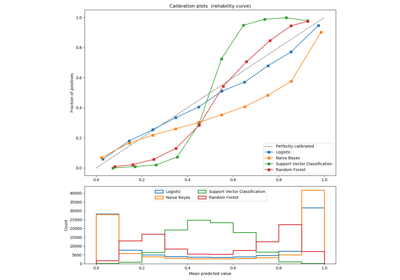Comparison of Calibration of Classifiers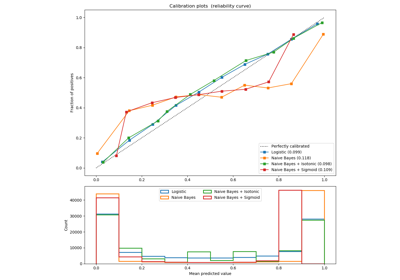Probability Calibration curves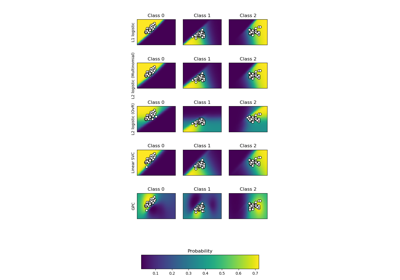Plot classification probabilityColumn Transformer with Mixed Types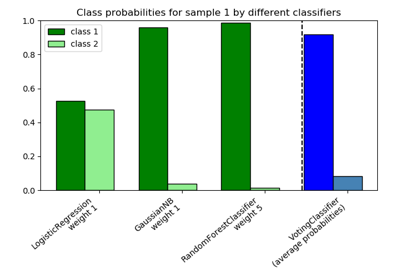Plot class probabilities calculated by the VotingClassifier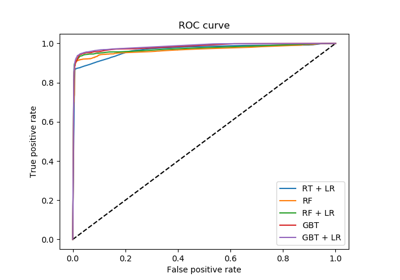Feature transformations with ensembles of treesDigits Classification Exercise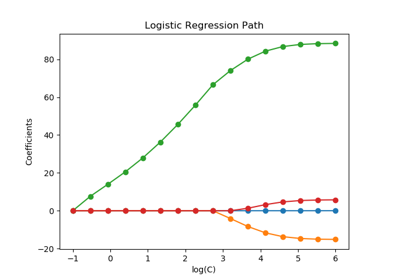Regularization path of L1- Logistic Regression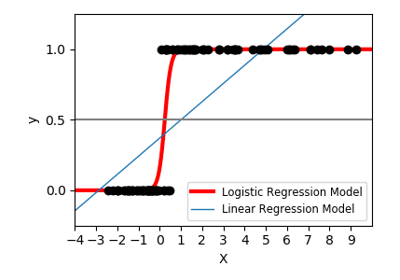Logistic function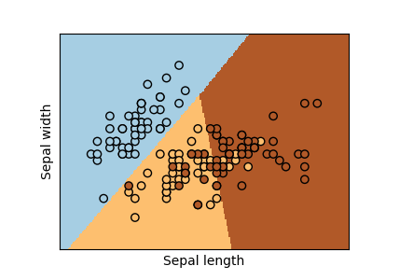Logistic Regression 3-class Classifier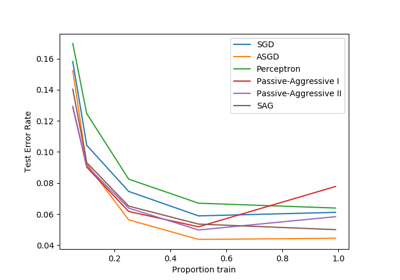Comparing various online solvers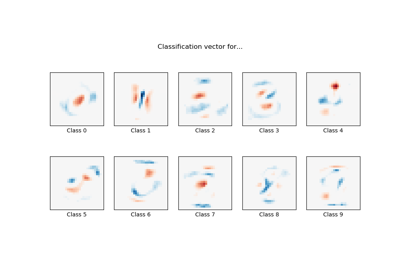MNIST classfification using multinomial logistic + L1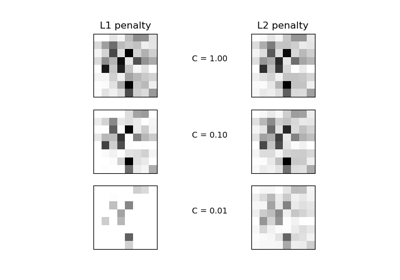L1 Penalty and Sparsity in Logistic Regression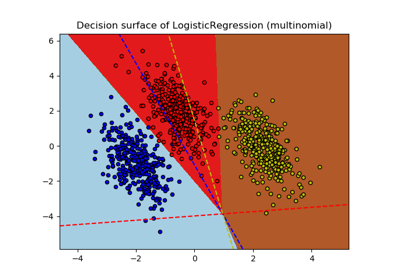Plot multinomial and One-vs-Rest Logistic Regression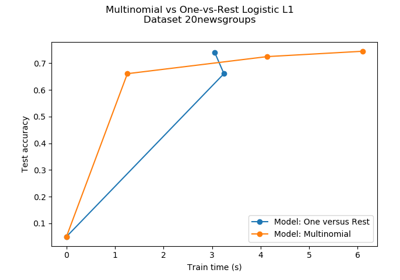Multiclass sparse logisitic regression on newgroups20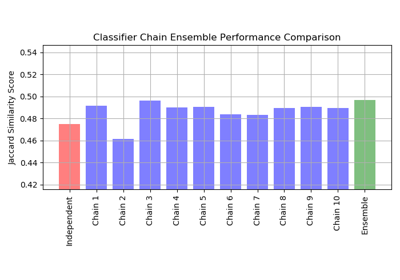Classifier Chain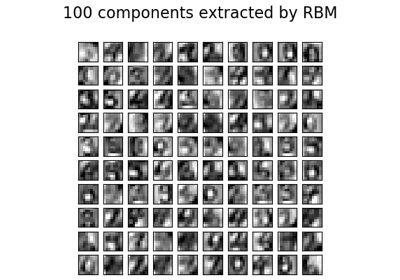Restricted Boltzmann Machine features for digit classification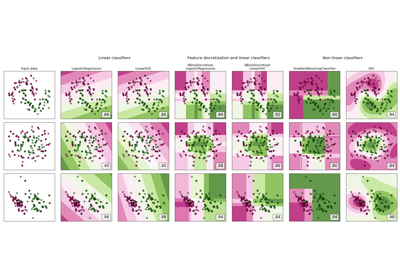Feature discretization